Blob Farm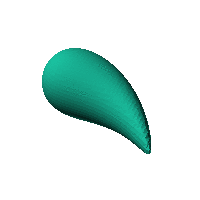# blob16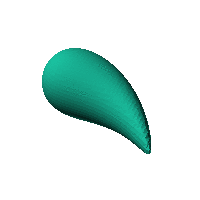The blob in all its glory: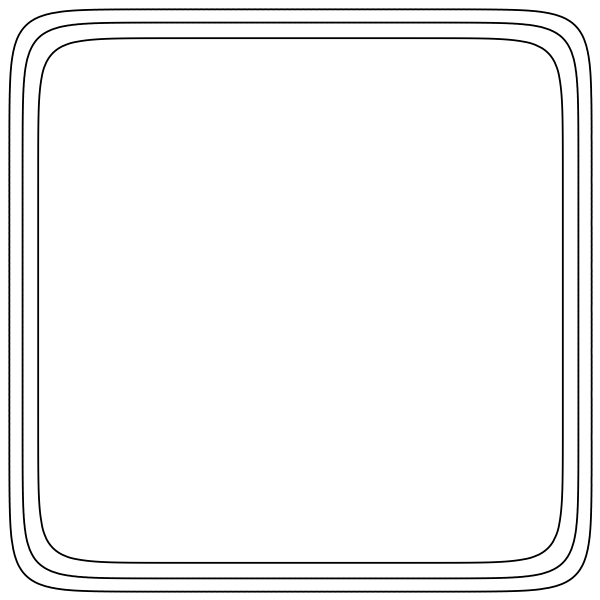Teardrop shape, good cat toy if sized well.

fx = 1.7*atan(x/1.7);
fy = y+0.03*x.^3;
fz = z;
sqrt(((fx-1)/1).^2+((fy-1)/1).^2+((fz-0)/1).^2) > 1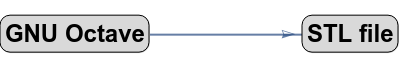Click on the snapshot to download the blob's stl file.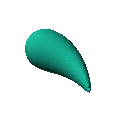Octave Code:
```1; # Prevent Octave from thinking that this is a function
# though one is defined here

function w = f(x2,y2,z2,c,r,e)
x  = (x2-c(1))/r(1);
y  = (y2-c(2))/r(2);
z  = (z2-c(3))/r(3);
# function at origin must be <0, and >0 far enough away.  w=0 defines the surface
fx = 1.7*atan(x/1.7);
fy = y+0.03*x.^3;
fz = z;
w = sqrt(((fx-1)/1).^2+((fy-1)/1).^2+((fz-0)/1).^2) -1;

endfunction;

```
GNU Octave